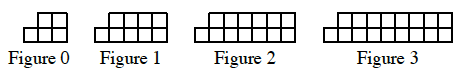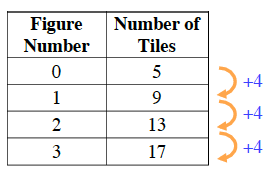### Home > AC > Chapter 4 > Lesson 4.1.4 > Problem4-35

4-35.

Examine the $x → y$ table below.

Figure Number

Number of Tiles

$0$

$5$

$1$

$9$

$2$

$13$

$3$

$17$

1. Invent a tile pattern that fits this data.

Possible Pattern2. What is the pattern’s growth factor? Show where the growth factor appears in the $x → y$ table and the tile pattern.Use the eTool below to help solve the problem.
Click the link at right for the full version of the eTool: 4-35 HW eTool (CPM)